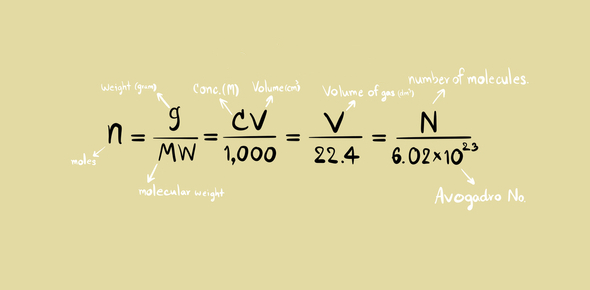# MCQs On Stoichiometry And Stoichiometric Calculations

18 Questions | Attempts: 736
ShareSettingsCheck out these MCQs on stoichiometry and stoichiometric calculations and see how good you're at using chemistry problems based on chemical formulas. Stoichiometry is a branch of chemistry that calculates various quantities of reactants and products of a chemical reaction using balanced chemical equations. How quickly can you solve a stoichiometric calculation accurately? Why don't you play the below quiz and see for yourself? Shall we begin the test? Best of luck, buddy!

• 1.
How many molecules are there in one mole of H2O?
• A.

6.023 x 1019

• B.

6.023 x 1023

• C.

1.084 x 1018

• D.

None of these

• 2.
• A.

40 g

• B.

100 g

• C.

50 g

• D.

1.427 g

• 3.
A necklace has 6 g of diamond in it. What are the number of atoms in it?
• A.

6.02 x 1023

• B.

12.04 x 1023

• C.

1.003 x 1023

• D.

3.01 x 1023

• 4.
What is the mass of aluminum in204 g of the aluminum oxide, Al2O3?
• A.

26 g

• B.

27 g

• C.

54 g

• D.

108 g

• 5.
The reactant which is consumed earlier and gives least quantity of product is called
• A.

Reactant in excess

• B.

Stoichiometry

• C.

Limiting reactant

• D.

Stoichiometric amount

• 6.
Which one of the following compounds contains the highest percentage by the mass of nitrogen
• A.

NH3

• B.

N2H4

• C.

NO

• D.

NH2OH

• 7.
Vitamin-A has a molecular formula C20H30O. The number of vitamin-A molecules in 500 mg of its capsule will be?
• A.

6.02 x 1023

• B.

1.05 x 1021

• C.

3.01 x 1022

• D.

3.01 x 1023

• 8.
When one mole of each of the following is completely burnt in oxygen, which will give the largest mass of CO2?
• A.

Carbon monoxide

• B.

Diamond

• C.

Ethane

• D.

Methane

• 9.
One mole of ethanol and one mole of ethane have an equal
• A.

Mass

• B.

Number of atoms

• C.

Number of electron

• D.

Number of molecules

• 10.
Methane react with steam to form H2 and CO as shown below. What volume of H can be obtained from 100 cmof methane at the standard temperature and pressure?
• A.

300 cm3

• B.

200 cm3

• C.

150 cm3

• D.

100 cm3

• 11.
The Avogadro's constant is the number of ?
• A.

Atoms in 1 g of Helium

• B.

Molecules in 35.5 g of chloride

• C.

Electrons needed to deposit 24 g of Mg

• D.

Atoms in 24 g of Mg

• 12.
How many mole of oxygen are needed for the complete combustion of two moles of butane?
• A.

2

• B.

8

• C.

10

• D.

13

• 13.
If four moles of SO2 are oxidized to SO, how many moles of oxygen molecules are required?
• A.

0.5

• B.

1.0

• C.

1.5

• D.

2.0

• 14.
What is the mass of 2 moles of chlorine gas if the relative atomic mass of chlorine is 35.5 amu?
• A.

142 g

• B.

71 g

• C.

35.5 g

• D.

18.75 g

• 15.
Which of the following statements is incorrect?
• A.

12 g of carbon gas contains one mole of atoms

• B.

28 g of nitrogen gas contains one mole of molecules of N2

• C.

1dmof 1.0 mole dm-3 solution of NaCl contains one mole of chloride ions

• D.

None of above

• 16.
One mole of propane has the same
• A.

Number of H- atoms as one mole of methane (CH4)

• B.

Number of C-atoms as in one mole of butane (C4H10)

• C.

Mass as half a mole of hexane (C6H14)

• D.

Number of molecules as in one mole of ethane (C2H6)

• 17.
What is the mass of one mole of iodine molecules?
• A.

254 g

• B.

74 g

• C.

106 g

• D.

127 g

• 18.
What volume of SO2 at room temperature and pressure is produced on heating 9.7 g of Zinc sulphide (ZnS) if reaction takes place as follows?
• A.

1.2 dm3

• B.

2.4 dm3

• C.

3.6 dm3

• D.

4.8 dm3

• E.

None of these

## Related TopicsBack to top
×

Wait!
Here's an interesting quiz for you.